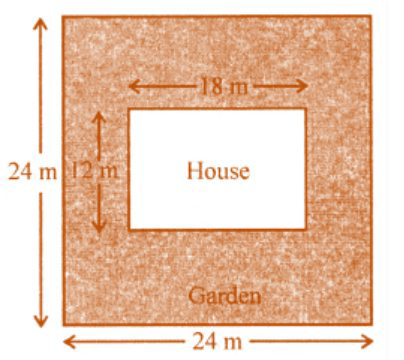# ML Aggarwal Solutions Class 8 Mathematics Solutions for Mensuration Exercise 18.1 in Chapter 18 - Mensuration

Rajesh has a square plot with the measurement as shown in the given figure. He wants to construct a house in the middle of the plot. A garden is developed around the house. Find the total cost of developing a garden around the house at the rate of ₹50 per m2.Given,

Side of square plot = 24 m

Length of house (l) = 18 m

Now,

Area of square plot = (24)2 m2 = (24 × 24) m2 = 576 m2

And,

Area of house = 18 × 12 = 216 m2

Remaining area of the garden = 576 m2 – 216 m2 = 360 m2

The cost of developing the garden = ₹50 per m2

Therefore, the total cost = ₹50 × 360 = ₹18000

Related Questions

Lido

Courses

Teachers

Book a Demo with us

Syllabus

Maths
CBSE
Maths
ICSE
Science
CBSE

Science
ICSE
English
CBSE
English
ICSE
Coding

Terms & Policies

Selina Question Bank

Maths
Physics
Biology

Allied Question Bank

Chemistry
Connect with us on social media!